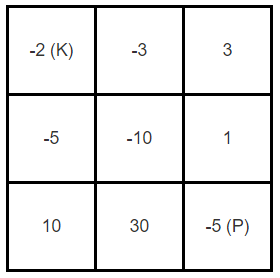174-dungeon-game

Question

The demons had captured the princess (P) and imprisoned her in the bottom-right corner of a dungeon. The dungeon consists of M x N rooms laid out in a 2D grid. Our valiant knight (K) was initially positioned in the top-left room and must fight his way through the dungeon to rescue the princess.

The knight has an initial health point represented by a positive integer. If at any point his health point drops to 0 or below, he dies immediately.

Some of the rooms are guarded by demons, so the knight loses health (negative integers) upon entering these rooms; other rooms are either empty (0's) or contain magic orbs that increase the knight's health (positive integers).

In order to reach the princess as quickly as possible, the knight decides to move only rightward or downward in each step.

Write a function to determine the knight's minimum initial health so that he is able to rescue the princess.

For example, given the dungeon below, the initial health of the knight must be at least7if he follows the optimal pathRIGHT-> RIGHT -> DOWN -> DOWN.

Example:Notes:

• The knight's health has no upper bound.
• Any room can contain threats or power-ups, even the first room the knight enters and the bottom-right room where the princess is imprisoned.

Thought Process

1. Dynamic Programing
1. To find the minimum hp require to rescue the princess, we need to find the hp required before entering the room
2. The hp depends on the dungeon[i][j], therefore we need to start from the right bottom of the array
3. The hp[i][j] = min(right, down) and right and down hp is equal to hp(right) - dungeon[i][j] or 1
4. Time complexity O(mn)
5. Space complexity O(mn)
2. Top Down Memo
1. We can use top down approach as well
2. For out of boundary search, we return integer max value because we want the min hp requirement
3. Once we got the min of right and down, we need to either further increase the hp requirement or reduce the hp requirement
4. If the dungeon gives more hp than the requirement, we can reset the hp requirement to 1
5. Time complexity O(mn)
6. Space complexity O(mn)

Solution

class Solution {
public int calculateMinimumHP(int[][] dungeon) {
if (dungeon.length == 0 || dungeon.length == 0) return 1;
int m = dungeon.length, n = dungeon.length;
int[][] hp = new int[m][n];
hp[m - 1][n - 1] = 1 - Math.min(dungeon[m - 1][n - 1], 0);
for (int j = n - 2; j >= 0; j--) {
hp[m - 1][j] = Math.max(hp[m - 1][j + 1] - dungeon[m - 1][j], 1);
}
for (int i = m - 2; i >= 0; i--) {
hp[i][n - 1] = Math.max(hp[i + 1][n - 1] - dungeon[i][n - 1], 1);
}
for (int i = m - 2; i >= 0; i--) {
for (int j = n - 2; j >= 0; j--) {
int down = Math.max(hp[i + 1][j] - dungeon[i][j], 1);
int right = Math.max(hp[i][j + 1] - dungeon[i][j], 1);
hp[i][j] = Math.min(down, right);
}
}
return hp;
}
}
class Solution {
public int calculateMinimumHP(int[][] dungeon) {
if (dungeon.length == 0 || dungeon.length == 0) return 1;
int m = dungeon.length, n = dungeon.length;
int[][] memo = new int[m][n];
memo[m - 1][n - 1] = 1 - Math.min(dungeon[m - 1][n - 1], 0);
return search(dungeon, 0, 0, memo);
}

private int search(int[][] dungeon, int i, int j, int[][] memo) {
if (i >= dungeon.length || j >= dungeon[i].length) return Integer.MAX_VALUE;
if (memo[i][j] != 0) return memo[i][j];
int right = search(dungeon, i, j + 1, memo);
int down = search(dungeon, i + 1, j, memo);
int hp = Math.min(right, down);
memo[i][j] = hp > dungeon[i][j] ? hp - dungeon[i][j] : 1;
return memo[i][j];
}
}